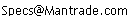Daily Speculations The Web Site of Victor Niederhoffer and Laurel KennerBernd Dittmann ContinuedWrite to us at:(address is not clickable)

Appendix: Data

Below are the percentage returns of the S&P500 on the trading day before and after US Independence Day.

```Yearbeforeafter
1950-0.2830.964
19510.6161.931
1952-0.04-0.319
19530.2060.082
19541.3011.115
19550.391.214
19560.8311.014
19571.1690.475
19580.3310.33
19590.5260.624
19600.246-0.07
19610.8820.644
19621.1280.566
19630.6910.4
19640.4010.46
19650.805-0.2
19661.0270.245
19670.2980.495
19681.1731.021
19690.677-0.582
1970-0.027-1.563
19710-0.02
19720.3270.567
1973-1.001-0.088
1974-0.059-0.7
19750.191-0.869
19760.502-0.547
1977-0.378-0.01
1978-0.461-0.862
19790.0980.333
19801.5390.707
1981-0.871-0.987
1982-0.975-0.334
19830.597-1.21
19840.326-0.612
1985-0.2920.559
1986-0.36-3.074
19870.888-0.232
1988-0.6291.483
19890.3930.442
19900.172-1.244
1991-1.0970.201
1992-0.2690.503
1993-0.708-0.989
19940.4340.038
19950.430.031
1996-0.18-2.225
19971.426-0.515
1998-0.1860.952
19990.743-0.223
20001.027-1.586
2001-0.184-1.232
20020.6223.673
2003-0.811.899
2004-0.315-0.815
20050.2610.883
20060.786-0.725

Appendix: Test results

Normality Tests

Anderson-Darling normality test

data:  before
A = 0.2114, p-value = 0.8502

Anderson-Darling normality test

data:  before[1:28]
A = 0.2028, p-value = 0.864

Anderson-Darling normality test

data:  before[29:57]
A = 0.2233, p-value = 0.8081

Anderson-Darling normality test

data:  after
A = 0.4453, p-value = 0.2737

Anderson-Darling normality test

data:  after[1:28]
A = 0.2168, p-value = 0.8265

Anderson-Darling normality test

data:  after[29:57]
A = 0.3407, p-value = 0.4719

Significance of returns: pre-ID returns

One Sample t-test

data:  before
t = 2.9518, df = 56, p-value = 0.004609
alternative hypothesis: true mean is not equal to 0
95 percent confidence interval:
0.08081405 0.42216841
sample estimates:
mean of x
0.2514912

One Sample t-test

data:  before[1:28]
t = 4.2417, df = 27, p-value = 0.0002329
alternative hypothesis: true mean is not equal to 0
95 percent confidence interval:
0.2199671 0.6321757
sample estimates:
mean of x
0.4260714

One Sample t-test

data:  before[29:57]
t = 0.6346, df = 28, p-value = 0.5308
alternative hypothesis: true mean is not equal to 0
95 percent confidence interval:
-0.1847453  0.3506074
sample estimates:
mean of x
0.08293103

Significance of returns: post-ID returns:

One Sample t-test

data:  after
t = 0.2483, df = 56, p-value = 0.8048
alternative hypothesis: true mean is not equal to 0
95 percent confidence interval:
-0.2502418  0.3210488
sample estimates:
mean of x
0.03540351

One Sample t-test

data:  after[1:28]
t = 1.8481, df = 27, p-value = 0.07556
alternative hypothesis: true mean is not equal to 0
95 percent confidence interval:
-0.0282567  0.5410424
sample estimates:
mean of x
0.2563929

One Sample t-test

data:  after[29:57]
t = -0.7346, df = 28, p-value = 0.4687
alternative hypothesis: true mean is not equal to 0
95 percent confidence interval:
-0.6742498  0.3183188
sample estimates:
mean of x
-0.1779655

correlations:

cor(before[1:28], after[1:28])
 0.4042747

cor(before[29:57], after[29:57])
 0.03629517

pre-'77 vs. post-'77

> t.test(before[1:28], before[29:57], equal.var=FALSE)

Welch Two Sample t-test

data:  before[1:28] and before[29:57]
t = 2.0819, df = 52.028, p-value = 0.04229
alternative hypothesis: true difference in means is not equal to 0
95 percent confidence interval:
0.01240652 0.67387427
sample estimates:
mean of x  mean of y
0.42607143 0.08293103

variances:

> var(before[1:28])
 0.28252

> var(before[29:57])
 0.4952054

> var(after[1:28])
 0.538885

> var(after[29:57])
 1.702262```